# Full Subtractor in Digital Logic

• Difficulty Level : Easy
• Last Updated : 21 Jun, 2022

A full subtractor is a combinational circuit that performs subtraction of two bits, one is minuend and other is subtrahend, taking into account borrow of the previous adjacent lower minuend bit. This circuit has three inputs and two outputs. The three inputs A, B and Bin, denote the minuend, subtrahend, and previous borrow, respectively. The two outputs, D and Bout represent the difference and output borrow, respectively. Although subtraction is usually achieved by adding the complement of subtrahend to the minuend, it is of academic interest to work out the Truth Table and logic realisation of a full subtractor; x is the minuend; y is the subtrahend; z is the input borrow; D is the difference; and B denotes the output borrow. The corresponding maps for logic functions for outputs of the full subtractor namely difference and borrow.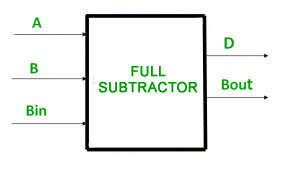Truth Table –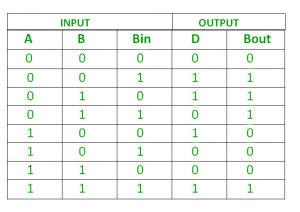From above table we can draw the K-Map as shown for “difference” and “borrow”.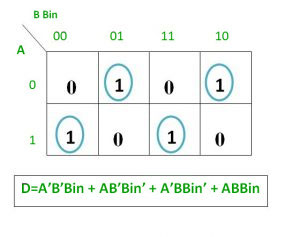Logical expression for difference –

```D   = A’B’Bin + A’BBin’ + AB’Bin’ + ABBin
= Bin(A’B’ + AB)  + Bin’(AB’ + A’B)
= Bin( A XNOR B) + Bin’(A XOR B)
= Bin (A XOR B)’  +  Bin’(A XOR B)
= Bin XOR (A XOR B)
= (A XOR B) XOR Bin```

Logical expression for borrow –

```Bout = A’B’Bin + A’BBin’ + A’BBin + ABBin
= A’B’Bin +A’BBin’ + A’BBin + A’BBin + A’BBin + ABBin
= A’Bin(B + B’) + A’B(Bin + Bin’) + BBin(A + A’)
= A’Bin + A’B + BBin

OR

Bout = A’B’Bin + A’BBin’ + A’BBin + ABBin
= Bin(AB + A’B’) + A’B(Bin + Bin’)
= Bin( A XNOR B) + A’B
= Bin (A XOR B)’ + A’B```

Logic Circuit for Full Subtractor –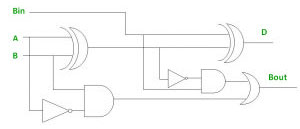Implementation of Full Subtractor using Half Subtractors – 2 Half Subtractors and an OR gate is required to implement a Full Subtractor.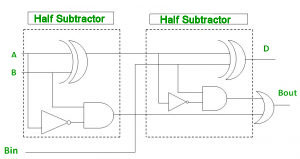My Personal Notes arrow_drop_up